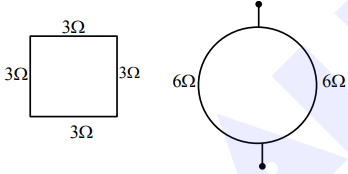# A square shaped wire with resistance of eachQuestion:

A square shaped wire with resistance of each side $3 \Omega$ is bent to form a complete circle. The resistance between two diametrically opposite points of the circle in unit of $\Omega$ will be_________.

Solution:$\mathrm{R}_{\mathrm{eq}}=3 \Omega$